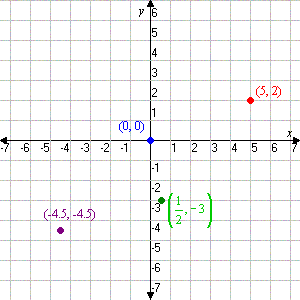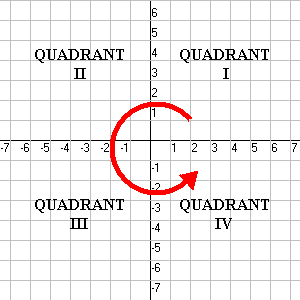# Plotting Points on a Cartesian Plane

A Cartesian plane (named after French mathematician Rene Descartes, who formalized its use in mathematics) is defined by two perpendicular number lines: the $x$-axis, which is horizontal, and the $y$-axis, which is vertical. Using these axes, we can describe any point in the plane using an ordered pair of numbers.

The Cartesian plane extends infinitely in all directions. To show this, math textbooks usually put arrows at the ends of the axes in their drawings.

The location of a point in the plane is given by its coordinates, a pair of numbers enclosed in parentheses: $\left(x,y\right)$. The first number $x$ gives the point's horizontal position and the second number $y$ gives its vertical position. All positions are measured relative to a "central" point called the origin, whose coordinates are $\left(0,0\right)$. For example, the point $\left(5,2\right)$ is $5$ units to the right of the origin and $2$ units up, as shown in the figure. Negative coordinate numbers tell us to go left or down. See the other points in the figure for examples.The Cartesian plane is divided into four quadrants. These are numbered from $\text{I}$ through $\text{IV}$, starting with the upper right and going around counterclockwise. (For some reason everybody uses roman numerals for this).In Quadrant $\text{I}$, both the $x$- and $y$-coordinates are positive; in Quadrant $\text{II}$, the $x$-coordinate is negative, but the $y$-coordinate is positive; in Quadrant $\text{III}$ both are negative; and in Quadrant IV $x$ is positive but $y$ is negative.

Points which lie on an axis (i.e., which have at least one coordinate equal to $0$) are said not to be in any quadrant. Coordinates of the form $\left(x,0\right)$ lie on the horizontal $x$-axis, and coordinates of the form $\left(0,y\right)$ lie on the vertical $y$-axis.